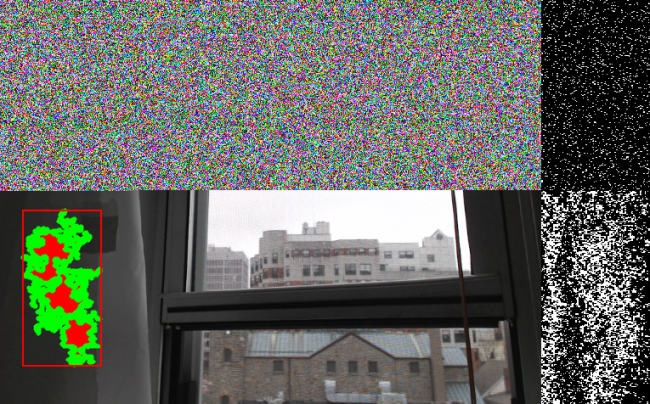#### Howdy, Stranger!

We are about to switch to a new forum software. Until then we have removed the registration on this forum.

# Voronoi 2D from OpenCV blob/ contour

edited April 2016

Hello: I am trying to create a surface mesh ultimately, from OpenCV detected contours using ToxicLibs. I first want to create the Voronoi2D points, then convert them to 3D and add them to a mesh. Or Simply Triangulate the contour as a plane using the Voronoi class, then add that plane as faces to a mesh.

However I am getting stuck on the best way to add the voronoi points, my program is slow and keeps crashing. This is because the contour and bounding box change every frame, so operations have to occur every frame. Using nested for loops seems like a bad idea given that, but I am not sure how else to do it. Please let me know if you have advice.

If I use only the PolygonApproximation Contour, I can get the voronoi points and not compromise speed (yet), but i am wondering what the best way to convert these to 3D faces would be next. Thanks.

Tagged:

•very frozen and slow.

• Hi all,

Here is almost the solution to this, though there is room for improvement!

``````       import gab.opencv.*;
import processing.video.*;
import java.awt.Rectangle;

import toxi.geom.*;
import toxi.geom.mesh.*;
import java.util.Iterator;

import toxi.physics.*;
import toxi.physics.behaviors.*;
import toxi.physics.constraints.*;
//for 2d

import toxi.geom.*;
import toxi.geom.mesh2d.*;

import toxi.util.*;
import toxi.util.datatypes.*;

import toxi.processing.*;

//// ranges for x/y positions of points
FloatRange xpos, ypos;

// helper class for rendering
ToxiclibsSupport gfx;

// empty voronoi mesh container
Voronoi voronoi = new Voronoi();

// optional polygon clipper
PolygonClipper2D clip;
Polygon2D polygon;

ArrayList triangles = new ArrayList();
ArrayList points = new ArrayList();
//for mesh/shape

int s;

WETriangleMesh mesh1;
VerletPhysics physics, emptyp;

PShape poly;

Vec3D prev=new Vec3D();
Vec3D p=new Vec3D();
Vec3D q=new Vec3D();

Vec2D newblob=new Vec2D();

float weight=0;
color c;
Capture video;
OpenCV opencv;
PImage src, colorFilteredImage;
ArrayList<Contour> contours;

// <1> Set the range of Hue values for our filter
int rangeLow = 20;
int rangeHigh = 35;

// radius of the root delaunay triangle which encompasses (MUST) all other points
float DELAUNAY_SIZE = 10000;

void setup() {
gfx = new ToxiclibsSupport(this);

String[] cameras = Capture.list();
println("Available cameras:");
//println(cameras);

video = new Capture(this, 640, 480, cameras);
video.start();

opencv = new OpenCV(this, video.width, video.height);
contours = new ArrayList<Contour>();

mesh1 = new WETriangleMesh("doodle");
size(900,900, P3D);
//smooth();

}

void draw() {

updatePixels();
if (video.available()) {
}
opencv.useColor();
src = opencv.getSnapshot();
opencv.useColor(HSB);
opencv.setGray(opencv.getH().clone());
opencv.inRange(rangeLow, rangeHigh);
colorFilteredImage = opencv.getSnapshot();
contours = opencv.findContours(true, true);
image(src, 0, 0);
image(colorFilteredImage, src.width, 0);

if (contours.size() > 0) {
//update voronoi per frame
voronoi = new Voronoi();
Contour biggestContour = contours.get(0);
Rectangle r = biggestContour.getBoundingBox();

//biggestCountour.
//draw other stuff
pushMatrix();
noFill();
strokeWeight(2);
stroke(255, 0, 0);
rect(r.x, r.y, r.width, r.height);
noStroke();
// fill(255, 0, 0);
ellipse(r.x + r.width/2, r.y + r.height/2, 30, 30);
biggestContour.setPolygonApproximationFactor(2);
stroke(100, 100, 100);
biggestContour.getPolygonApproximation().draw();
//biggestContour.draw();
Contour ApproxContour =biggestContour.getPolygonApproximation();

popMatrix();

//for (Vertex v : mesh1.vertices.values()) {
//v.set(physics.particles.get(v.id)); }

//convert countour to points
for (PVector point : ApproxContour.getPoints()) {
//vertex(point.x, point.y, point.z);
println("points:", point.x, ",", point.y);
}

for (Vec2D c : voronoi.getSites()) {
//fill(0, 255, 0);
ellipse(c.x, c.y, 5, 5);
//for each voronoi site, create a vec 3d physics particle
physics = new VerletPhysics();
//for (Vertex v : mesh1.vertices.values()) {

}

println("particles:",  physics.particles);
updateMesh(mesh1);
smooth();
drawMesh(r.x +r.width/2, r.y+r.height/2, -0.3);
Clear();

}

}

void resetMesh(WETriangleMesh TheMesh, VerletPhysics P) {

P.update();
for (Vertex v : TheMesh.vertices.values()) {
v.set(P.particles.get(v.id));
}
TheMesh.center(null);
for (Vertex v : TheMesh.vertices.values()) {
P.particles.get(v.id).set(v);
}
TheMesh.computeFaceNormals();
TheMesh.faceOutwards();
TheMesh.computeVertexNormals();

}

void Clear() {
mesh1.clear();

}

void initphyiscs() {

}

void getTexture() {
}

void updateMesh(WETriangleMesh mesh) {
mesh=new WETriangleMesh();

for (Triangle2D t : voronoi.getTriangles()) {
//noFill();
//gfx.triangle(t, false);
// endShape();
//   t.computeCentroid();  }

Vec3D a = new Vec3D((t.a.x), (t.a.y), 0);
//Vec3D b = new Vec3D((t.a.x), 0, (t.a.y));

if (abs(a.x)!=10000 && abs(a.y)!=10000) {
println("faces", "-", a, "-");

// turn mesh vertices into physics particles
}
}

/*  for(VerletParticle i : physics.particles) {
VerletParticle a=physics.particles.get(i);
VerletParticle b=physics.particles.get(i+1);
VerletParticle c=physics.particles.get(i+1+DIM);
VerletParticle d=physics.particles.get(i+DIM);
}
*/
}

void vertex(Vec3D v) {
vertex(v.x,v.y,v.z);
}

void drawMesh(int x, int y, float s) {

mesh1.subdivide();
//mesh1.subdivide();
mesh1.computeFaceNormals();
mesh1.faceOutwards();
mesh1.computeVertexNormals();
mesh1.center(null);

//mesh1.subdivide();
pushMatrix();
// background(0);
lights();
scale(1);
translate(x, y, 0);
//rotateY((width / 2 - mouseX) * 0.01f);
//rotateY((width / 2 - mouseY) * 0.01f);
//rotateY((width / 2 - mouseX) * 0.01f);

noStroke();
fill(200, 10, 10);
//stroke(10, 10, 200);
//strokeWeight(1);
gfx.mesh(mesh1, true, 0);
popMatrix();

}

void mousePressed() {

color c = get(mouseX, mouseY);
println("r: " + red(c) + " g: " + green(c) + " b: " + blue(c));

int hue = int(map(hue(c), 0, 255, 0, 180));
println("hue to detect: " + hue);

rangeLow = hue - 5;
rangeHigh = hue + 5;
}

void keyPressed() {
if (key=='s') {
mesh1.saveAsOBJ(sketchPath("doodle.obj"));
mesh1.saveAsSTL(sketchPath("doodle.stl"));
}
else {
mesh1.clear();
}
}

void AddPoint(int x, int y, Contour c) {
//updatePixels();
for ( int i = 0; i < x; i++) {
for ( int j = 0; j < y;j++) {
//int loc = i + j* x;

if (c.containsPoint(i, j)) {
fill(0, 0, 255);
ellipse(i, j, 2, 2);
println("gotpoint");
//println("point: (", i,",", j, ")");
//updatePixels();

//pick a random point
//int x = int(random(r.width));
// int y = int(random(r.height));

}

}

}

}
}

}`
``````